# scipy.spatial.distance.kulsinski¶

scipy.spatial.distance.kulsinski(u, v)
Computes the Kulsinski dissimilarity between two boolean n-vectors

u and v, which is defined asrac{c_{TF} + c_{FT} - c_{TT} + n}
{c_{FT} + c_{TF} + n}

where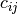is the number of occurrences of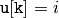and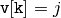for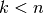.

Parameters : u : ndarray An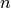-dimensional vector. v : ndarray An-dimensional vector. d : double The Kulsinski distance between vectors u and v.

#### Previous topic

scipy.spatial.distance.jaccard

#### Next topic

scipy.spatial.distance.mahalanobis